# Dynamic Memory Allocation in C using malloc(), calloc(), free() and realloc()

• Difficulty Level : Easy
• Last Updated : 09 Dec, 2021

Since C is a structured language, it has some fixed rules for programming. One of them includes changing the size of an array. An array is a collection of items stored at contiguous memory locations.As it can be seen that the length (size) of the array above made is 9. But what if there is a requirement to change this length (size). For Example,

• If there is a situation where only 5 elements are needed to be entered in this array. In this case, the remaining 4 indices are just wasting memory in this array. So there is a requirement to lessen the length (size) of the array from 9 to 5.
• Take another situation. In this, there is an array of 9 elements with all 9 indices filled. But there is a need to enter 3 more elements in this array. In this case, 3 indices more are required. So the length (size) of the array needs to be changed from 9 to 12.

This procedure is referred to as Dynamic Memory Allocation in C.
Therefore, C Dynamic Memory Allocation can be defined as a procedure in which the size of a data structure (like Array) is changed during the runtime.
C provides some functions to achieve these tasks. There are 4 library functions provided by C defined under <stdlib.h> header file to facilitate dynamic memory allocation in C programming. They are:

1. malloc()
2. calloc()
3. free()
4. realloc()

Let’s look at each of them in greater detail.

### C malloc() method

The “malloc” or “memory allocation” method in C is used to dynamically allocate a single large block of memory with the specified size. It returns a pointer of type void which can be cast into a pointer of any form. It doesn’t Initialize memory at execution time so that it has initialized each block with the default garbage value initially.

Syntax:

```ptr = (cast-type*) malloc(byte-size)
For Example:```

ptr = (int*) malloc(100 * sizeof(int));
Since the size of int is 4 bytes, this statement will allocate 400 bytes of memory. And, the pointer ptr holds the address of the first byte in the allocated memory.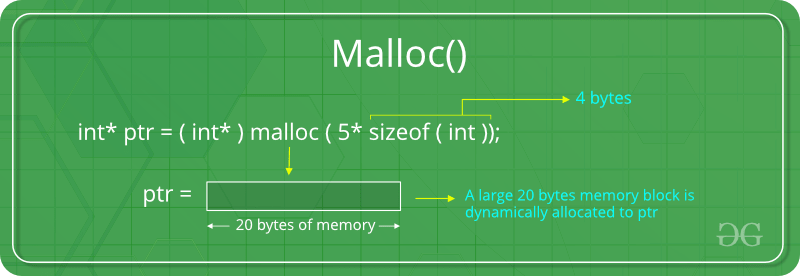If space is insufficient, allocation fails and returns a NULL pointer.

Example:

## C

 `#include ` `#include `   `int` `main()` `{`   `    ``// This pointer will hold the` `    ``// base address of the block created` `    ``int``* ptr;` `    ``int` `n, i;`   `    ``// Get the number of elements for the array` `    ``printf``(``"Enter number of elements:"``);` `    ``scanf``(``"%d"``,&n);` `    ``printf``(``"Entered number of elements: %d\n"``, n);`   `    ``// Dynamically allocate memory using malloc()` `    ``ptr = (``int``*)``malloc``(n * ``sizeof``(``int``));`   `    ``// Check if the memory has been successfully` `    ``// allocated by malloc or not` `    ``if` `(ptr == NULL) {` `        ``printf``(``"Memory not allocated.\n"``);` `        ``exit``(0);` `    ``}` `    ``else` `{`   `        ``// Memory has been successfully allocated` `        ``printf``(``"Memory successfully allocated using malloc.\n"``);`   `        ``// Get the elements of the array` `        ``for` `(i = 0; i < n; ++i) {` `            ``ptr[i] = i + 1;` `        ``}`   `        ``// Print the elements of the array` `        ``printf``(``"The elements of the array are: "``);` `        ``for` `(i = 0; i < n; ++i) {` `            ``printf``(``"%d, "``, ptr[i]);` `        ``}` `    ``}`   `    ``return` `0;` `}`

Output:

```Enter number of elements: 5
Memory successfully allocated using malloc.
The elements of the array are: 1, 2, 3, 4, 5,```

### C calloc() method

1. “calloc” or “contiguous allocation” method in C is used to dynamically allocate the specified number of blocks of memory of the specified type. it is very much similar to malloc() but has two different points and these are:
2. It initializes each block with a default value ‘0’.
3. It has two parameters or arguments as compare to malloc().

Syntax:

```ptr = (cast-type*)calloc(n, element-size);
here, n is the no. of elements and element-size is the size of each element.```

For Example:

ptr = (float*) calloc(25, sizeof(float));
This statement allocates contiguous space in memory for 25 elements each with the size of the float.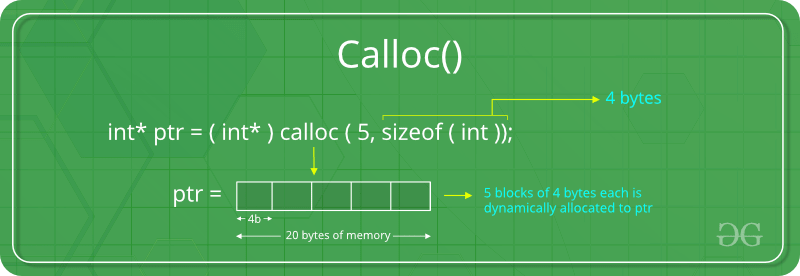If space is insufficient, allocation fails and returns a NULL pointer.

Example:

## C

 `#include ` `#include `   `int` `main()` `{`   `    ``// This pointer will hold the` `    ``// base address of the block created` `    ``int``* ptr;` `    ``int` `n, i;`   `    ``// Get the number of elements for the array` `    ``n = 5;` `    ``printf``(``"Enter number of elements: %d\n"``, n);`   `    ``// Dynamically allocate memory using calloc()` `    ``ptr = (``int``*)``calloc``(n, ``sizeof``(``int``));`   `    ``// Check if the memory has been successfully` `    ``// allocated by calloc or not` `    ``if` `(ptr == NULL) {` `        ``printf``(``"Memory not allocated.\n"``);` `        ``exit``(0);` `    ``}` `    ``else` `{`   `        ``// Memory has been successfully allocated` `        ``printf``(``"Memory successfully allocated using calloc.\n"``);`   `        ``// Get the elements of the array` `        ``for` `(i = 0; i < n; ++i) {` `            ``ptr[i] = i + 1;` `        ``}`   `        ``// Print the elements of the array` `        ``printf``(``"The elements of the array are: "``);` `        ``for` `(i = 0; i < n; ++i) {` `            ``printf``(``"%d, "``, ptr[i]);` `        ``}` `    ``}`   `    ``return` `0;` `}`

Output:

```Enter number of elements: 5
Memory successfully allocated using calloc.
The elements of the array are: 1, 2, 3, 4, 5,```

### C free() method

“free” method in C is used to dynamically de-allocate the memory. The memory allocated using functions malloc() and calloc() is not de-allocated on their own. Hence the free() method is used, whenever the dynamic memory allocation takes place. It helps to reduce wastage of memory by freeing it.

Syntax:

`free(ptr);`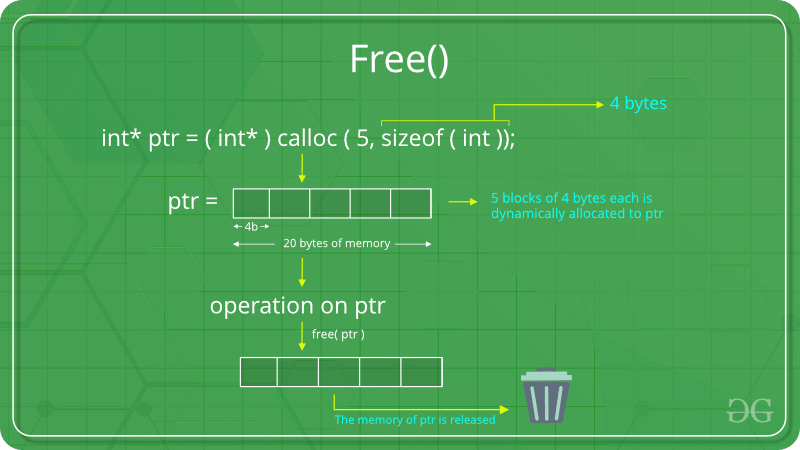Example:

## C

 `#include ` `#include `   `int` `main()` `{`   `    ``// This pointer will hold the` `    ``// base address of the block created` `    ``int` `*ptr, *ptr1;` `    ``int` `n, i;`   `    ``// Get the number of elements for the array` `    ``n = 5;` `    ``printf``(``"Enter number of elements: %d\n"``, n);`   `    ``// Dynamically allocate memory using malloc()` `    ``ptr = (``int``*)``malloc``(n * ``sizeof``(``int``));`   `    ``// Dynamically allocate memory using calloc()` `    ``ptr1 = (``int``*)``calloc``(n, ``sizeof``(``int``));`   `    ``// Check if the memory has been successfully` `    ``// allocated by malloc or not` `    ``if` `(ptr == NULL || ptr1 == NULL) {` `        ``printf``(``"Memory not allocated.\n"``);` `        ``exit``(0);` `    ``}` `    ``else` `{`   `        ``// Memory has been successfully allocated` `        ``printf``(``"Memory successfully allocated using malloc.\n"``);`   `        ``// Free the memory` `        ``free``(ptr);` `        ``printf``(``"Malloc Memory successfully freed.\n"``);`   `        ``// Memory has been successfully allocated` `        ``printf``(``"\nMemory successfully allocated using calloc.\n"``);`   `        ``// Free the memory` `        ``free``(ptr1);` `        ``printf``(``"Calloc Memory successfully freed.\n"``);` `    ``}`   `    ``return` `0;` `}`

Output:

```Enter number of elements: 5
Memory successfully allocated using malloc.
Malloc Memory successfully freed.

Memory successfully allocated using calloc.
Calloc Memory successfully freed.```

### C realloc() method

“realloc” or “re-allocation” method in C is used to dynamically change the memory allocation of a previously allocated memory. In other words, if the memory previously allocated with the help of malloc or calloc is insufficient, realloc can be used to dynamically re-allocate memory. re-allocation of memory maintains the already present value and new blocks will be initialized with the default garbage value.

Syntax:

```ptr = realloc(ptr, newSize);

where ptr is reallocated with new size 'newSize'.```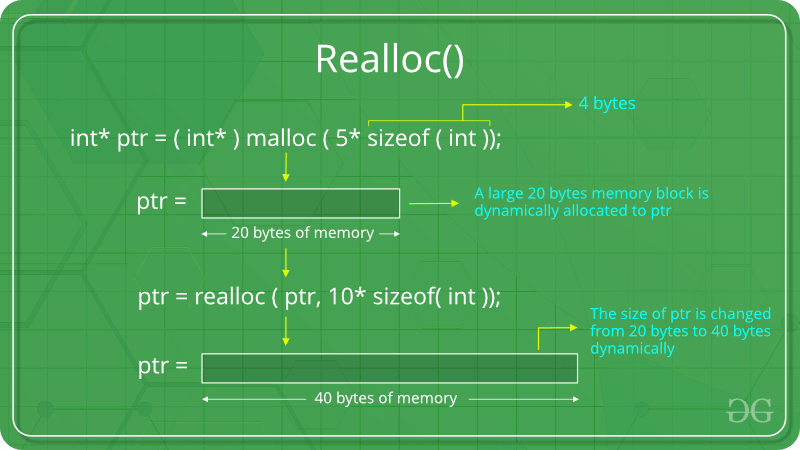If space is insufficient, allocation fails and returns a NULL pointer.

Example:

## C

 `#include ` `#include `   `int` `main()` `{`   `    ``// This pointer will hold the` `    ``// base address of the block created` `    ``int``* ptr;` `    ``int` `n, i;`   `    ``// Get the number of elements for the array` `    ``n = 5;` `    ``printf``(``"Enter number of elements: %d\n"``, n);`   `    ``// Dynamically allocate memory using calloc()` `    ``ptr = (``int``*)``calloc``(n, ``sizeof``(``int``));`   `    ``// Check if the memory has been successfully` `    ``// allocated by malloc or not` `    ``if` `(ptr == NULL) {` `        ``printf``(``"Memory not allocated.\n"``);` `        ``exit``(0);` `    ``}` `    ``else` `{`   `        ``// Memory has been successfully allocated` `        ``printf``(``"Memory successfully allocated using calloc.\n"``);`   `        ``// Get the elements of the array` `        ``for` `(i = 0; i < n; ++i) {` `            ``ptr[i] = i + 1;` `        ``}`   `        ``// Print the elements of the array` `        ``printf``(``"The elements of the array are: "``);` `        ``for` `(i = 0; i < n; ++i) {` `            ``printf``(``"%d, "``, ptr[i]);` `        ``}`   `        ``// Get the new size for the array` `        ``n = 10;` `        ``printf``(``"\n\nEnter the new size of the array: %d\n"``, n);`   `        ``// Dynamically re-allocate memory using realloc()` `        ``ptr = ``realloc``(ptr, n * ``sizeof``(``int``));`   `        ``// Memory has been successfully allocated` `        ``printf``(``"Memory successfully re-allocated using realloc.\n"``);`   `        ``// Get the new elements of the array` `        ``for` `(i = 5; i < n; ++i) {` `            ``ptr[i] = i + 1;` `        ``}`   `        ``// Print the elements of the array` `        ``printf``(``"The elements of the array are: "``);` `        ``for` `(i = 0; i < n; ++i) {` `            ``printf``(``"%d, "``, ptr[i]);` `        ``}`   `        ``free``(ptr);` `    ``}`   `    ``return` `0;` `}`

Output:

```Enter number of elements: 5
Memory successfully allocated using calloc.
The elements of the array are: 1, 2, 3, 4, 5,

Enter the new size of the array: 10
Memory successfully re-allocated using realloc.
The elements of the array are: 1, 2, 3, 4, 5, 6, 7, 8, 9, 10,```

One another example for realloc() method is:

## C

 `#include ` `#include ` `int` `main()` `{` `    ``int` `index = 0, i = 0, n,` `        ``*marks; ``// this marks pointer hold the base address` `                ``// of  the block created` `    ``int` `ans;` `    ``marks = (``int``*)``malloc``(``sizeof``(` `        ``int``)); ``// dynamically allocate memory using malloc` `    ``// check if the memory is successfully allocated by` `    ``// malloc or not?` `    ``if` `(marks == NULL) {` `        ``printf``(``"memory cannot be allocated"``);` `    ``}` `    ``else` `{` `        ``// memory has successfully allocated` `        ``printf``(``"Memory has been successfully allocated by "` `               ``"using malloc\n"``);` `        ``printf``(``"\n marks = %pc\n"``,` `               ``marks); ``// print the base or beginning` `                       ``// address of allocated memory` `        ``do` `{` `            ``printf``(``"\n Enter Marks\n"``);` `            ``scanf``(``"%d"``, &marks[index]); ``// Get the marks` `            ``printf``(``"would you like to add more(1/0): "``);` `            ``scanf``(``"%d"``, &ans);`   `            ``if` `(ans == 1) {` `                ``index++;` `                ``marks = (``int``*)``realloc``(` `                    ``marks,` `                    ``(index + 1)` `                        ``* ``sizeof``(` `                            ``int``)); ``// Dynamically reallocate` `                                   ``// memory by using realloc` `                ``// check if the memory is successfully` `                ``// allocated by realloc or not?` `                ``if` `(marks == NULL) {` `                    ``printf``(``"memory cannot be allocated"``);` `                ``}` `                ``else` `{` `                    ``printf``(``"Memory has been successfully "` `                           ``"reallocated using realloc:\n"``);` `                    ``printf``(` `                        ``"\n base address of marks are:%pc"``,` `                        ``marks); ``////print the base or` `                                ``///beginning address of` `                                ``///allocated memory` `                ``}` `            ``}` `        ``} ``while` `(ans == 1);` `        ``// print the marks of the students` `        ``for` `(i = 0; i <= index; i++) {` `            ``printf``(``"marks of students %d are: %d\n "``, i,` `                   ``marks[i]);` `        ``}` `        ``free``(marks);` `    ``}` `    ``return` `0;` `}`

Output: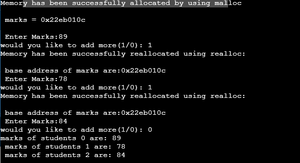My Personal Notes arrow_drop_up
Recommended Articles
Page :